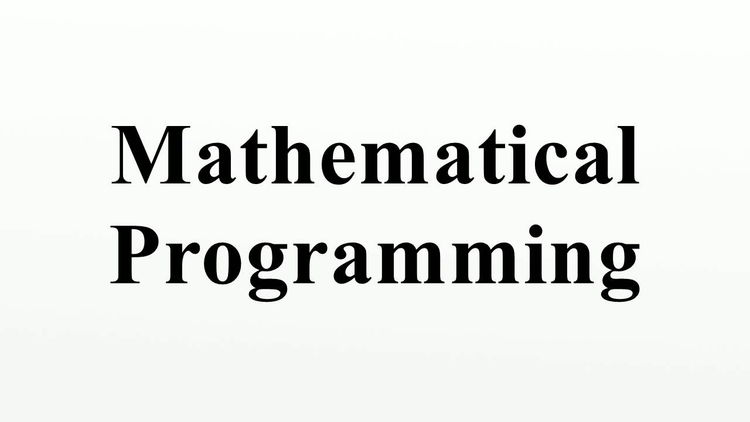## Introduction

You will have been assigned to this module as a condition of your acceptance to one of programmes.This booklet contains a combination of guides and the module activities. There are a total of nine activities for you to complete and submit for this module. You should read through each guide before attempting the activities.
You will have been assigned to this module as a condition of your acceptance to one of programmes.
This booklet contains a combination of guides and the module activities. There are a total of nine activities for you to complete and submit for this module. You should read through each guide before attempting the activities.

### Decimal Guide

A decimal is a way of writing a number that is not whole. A decimal number is in-between numbers.

For example, 7.465 are in between the numbers 7 and 8. So, it is more than 7, but less than 8.

Value headings that is tenths, hundredths etc. can be used to discover exactly what a decimal number represents.

Units    Tenths    Hundredths Thousandths 7 .               4      6      5

The numbers to the left of the decimal point are normal whole numbers. The numbers to the right of the decimal point are parts of whole numbers.

#### Rounding

It can be difficult to read and work with strings of numbers in a decimal fraction.  Rounding makes this easier to manage.

So, however many decimal places we are rounding to we decide the value of the last rounded digit based on the subsequent digits –if these themselves round to give a digit less than 5 then we round down, if these themselves round to give a digit greater than 5 then we round up as in the following examples: 3.457 to 2dp (decimal places) = 3.46

#### Long Division Guide

Below you will find a basic ‘how to’ guide to refresh your skills in long division. No remainders should be left in your long division for this activity; you must continue using decimal point.

There are a number of common ways to show division. 5717 divided by 13 could be written as: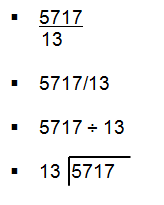All these are correct ways of expressing this division sum.

#### Fractions Guide

A fraction is part of a whole. In a numeracy context, a fraction equals one of a number of parts which complete a whole unit. For example: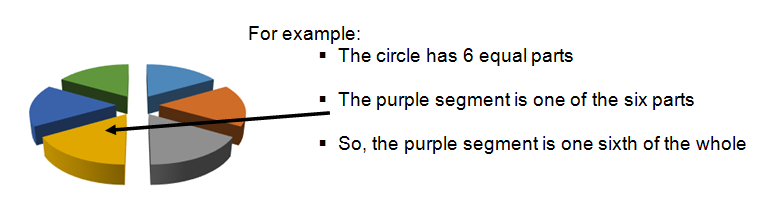Written Fractions

The top number of the fraction is known as the numeratorand represents the proportion of the equal parts into which the whole is divided.
The bottom number of the fraction is known as the denominatorand represents the total number of parts into which the whole is
divided.
For the example above, the purple segment would be shown as 1/6, one segment of six segments making up the whole unit.
If we want to represent all the segments except the purple one as a fraction this would be shown as 5/6, five segments of six segments making up the whole.

Proper and Improper Fractions

In a proper fraction the numeratoris smaller than the denominator. So, 1/6 and 5/6 would both be proper fractions.In an improper fraction the numerator is bigger than the denominator.So, 7/6 and 10/6 would both be improper fractions. This means the fraction is greater than 1.

A mixed fraction combines a whole number and a proper fraction. So the improper fractions above could also be written as 11/6 and 14/6.

Comparing Fractions

When fractions have the same denominator it is easy to compare them. For example let’s compare 1/6 and 5/6

By looking at the numerators we can tell that 5 is more than 1. Therefore 5/6 is larger than 1/6.

When the denominators differ, for example 1/6 and 3/5 you need to find a common denominator or which can be divided by both 6 and 5.

The easiest way to do this is multiply 6 x 5 = 30.

Therefore the new denominator will be 30

Next you need to work out the equivalent number of 1/30 for each fraction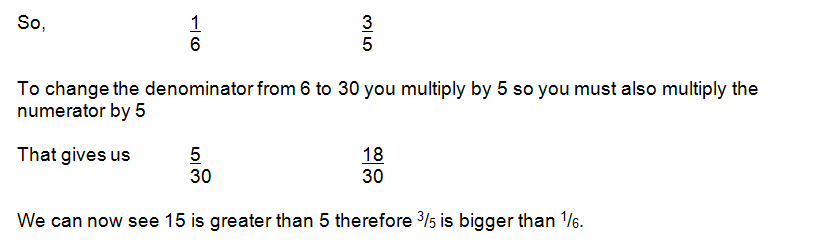Simplifying Fractions

When simplifying a fraction the numerator and denominator must be divided by the same value at the same time.

For Example:

20/30 Both are divisible by 10 so simplified this would be 2/3

2/6 both are divisible by 2 so simplified this would be 1/3

Converting Fractions to Decimals

To convert a fraction into a decimal we divide the numerator (top number) by the denominator (bottom number).

#### Volume

When we MULTIPLY by numbers like 10 or 100 or 1000 we move the point to the

RIGHT by the same number of places as there are zeros in the multiplier. e.g.          2.3 x 100 = 230             58 x 1000 = 58 000       0.06 x 10 = 0.6

When we DIVIDE by such numbers we move the point LEFT by the same number of places.

e.g.    43.7 ÷ 10 = 4.37            360 ÷ 100 = 3.60           9.5 ÷ 1000 = 0.0095

With volumes we require also two other measures. These are used quite extensively every day on the continent

For our nursing studies we require both decilitres (dL) and centilitres (cL). Here is the complete conversion chart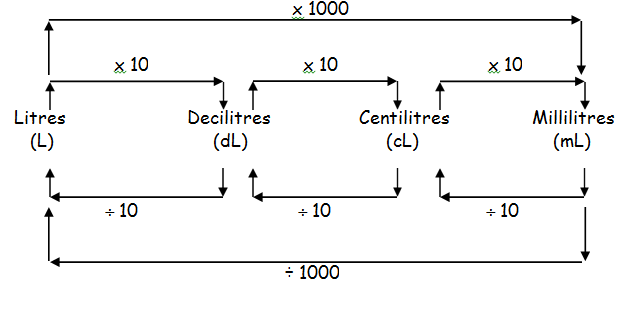Remember that  deci…means “one tenth of …"

centi…means “one hundredth of …”

milli…means “one thousandth of …”

It is much easier to measure accurately very small weights, than it is to measure volumes.  So we have names for weights smaller than the basic gram.You’ll probably know the first one.

1 milligram = one thousandth of a gram. We can write the relationship in several different ways:

1mg = 0.001g 1g = 1000mg

You may not have heard of the next smallest one.

1 microgram = one millionth of a gram. This makes it one thousandth of a milligram.  The symbol used in science for the prefix “micro” is m.

Thus 1000mg = 1mg. However, written quickly, or sloppily, this symbol m can look like the letter m, and cause confusion and possible danger, since a mg is 1000 times the size of a mg.

So we will use “mcg” for microgram.

Scientists use even smaller units (prefixed “nano” and “pico”) but you won’t need them.

#### Percentage Guide

Percent means per hundred or out of a hundred and the symbol for percent is %.

So if 34% of the population are over 60 years of age it means that 34 per hundred of the population are over 60 years of age.

An essential principle is that 100% means the whole number.100 – 34 = 66 so it can be established that since 34% of the population are over the age of 60 then 66% are not over the age of 60.

34% can be written as  34/100  therefore a percentage is essentially a fraction with 100 as the denominator. When appropriate this fraction can be simplified and/or expressed as a decimal.

Converting percentages to decimals

To convert a percentage to a decimal we are simply dividing by 100 7% = 7/100

This can be done by moving the decimal point two places to the left. Remember adding in zeros does not alter the number but assists us with our calculation.

007.0 /100 = 0.07

Converting fractions to percentages

To convert a fraction to a percentage we multiply by 100. A whole number can be written as fraction by placing it over 1.

1/8*100

= 1/8*100/1

=100/8=12.5%

Percentages are used as a standard method of expressing proportions of numbers or quantities which makes comparisons and our idea of the size easier.

Calculating percentage of a quantity

To calculate we multiply the number by the percentage required. What is 30% of 96?

30/100*96

=2880/100

=28.8